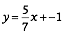# Riding the Berms

## OBJECTIVE: To learn how to graph a line on the Cartesian plane using the slope and the y-intercept

In a previous lesson, you were introduced to the STANDARD FORM of a line (Ax + By = C) and its relative, the GENERAL FORM (Ax + By + C = 0). When asked to find the equation of a line, you usually give your final answer in these forms. Drawing the Line: https://www.geogebra.org/m/bb3cpjrn In this lesson, you'll be introduced to another form of the equation of a line in which the final answer can be written—the SLOPE-INTERCEPT FORM (y = mx + b). Interact with the applet below to discover some facts about the SLOPE-INTERCEPT FORM.

## INSTRUCTIONS

1. Set slider m = 5 and slider b = 5. 2. Keep slider m = 5 and move slider b. Observe the movement of the graph. 3. Keep slider b = 5 and move slider m. Observe the movement of the graph. 4. Try other combinations of m and b. Observe how the graph behaves.

QUESTION: What happens to the graph when you move slider b while keeping slider m fixed?

QUESTION: What happens to the graph when you move slider m while keeping slider b fixed?

QUESTION: What do m and b represent in the formula: y = mx + b.

## INSTRUCTIONS

Use the applet below to complete the following table. Click "Show Hint" for the values of rise and run. The first has been done for you. Points Rise/Run Where Line Crosses Y-Axis Equation A (0, -1), (7, 4) ___5/7___ ___(0, -1)__ ________ B (0, -4), (6, 3) _________ __________ ________________ C (5, 1), (0, 4) _________ __________ ________________ D ﻿ (-4, -1), (0, -3) _________ __________ ________________ E ﻿ (0, 4), (2, 0) _________ __________ ________________ F ﻿ (0, 3), (8, 3) _________ __________ ________________ G ﻿(-5, 5), (0, -1) _________ __________ ________________ H (0, 0), (2, 2) _________ __________ ________________ I (0, 7), (-2, 5) _________ __________ ________________ J (-3, -4), (0, -6) _________ __________ ________________

QUESTION: ﻿For each row, do you notice any relationship among the values of rise/run, the point where the line crosses the y-axis, and the equation of the line? Briefly explain.

Interact with the applet below to practice graphing lines using the slope and the y-intercept. If your graph is correct, a big "Correct!!!" sign will appear. Otherwise, you'll have to try again. Repeat as many times as needed to master the concept.

## TODAY you were introduced to the SLOPE-INTERCEPT FORM of the equation of a line.

You discovered some of its properties, and you learned how to use the slope and the y-intercept to graph the line on the Cartesian plane. In future lessons, you'll discover more interesting facts about lines. Did you ENJOY your experience?